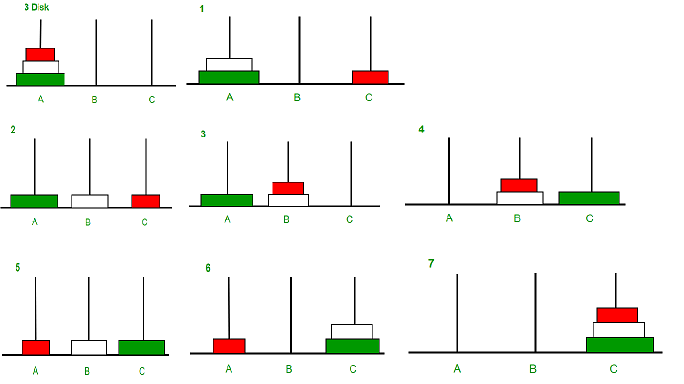# Java Program for Tower of Hanoi

Tower of Hanoi is a mathematical puzzle where we have three rods and n disks. The objective of the puzzle is to move the entire stack to another rod, obeying the following simple rules:
1) Only one disk can be moved at a time.
2) Each move consists of taking the upper disk from one of the stacks and placing it on top of another stack i.e. a disk can only be moved if it is the uppermost disk on a stack.
3) No disk may be placed on top of a smaller disk.## Java

 `// Java recursive program to solve tower of hanoi puzzle ` ` `  `class` `GFG ` `{ ` `    ``// Java recursive function to solve tower of hanoi puzzle ` `    ``static` `void` `towerOfHanoi(``int` `n, ``char` `from_rod, ``char` `to_rod, ``char` `aux_rod) ` `    ``{ ` `        ``if` `(n == ``1``) ` `        ``{ ` `            ``System.out.println(``"Move disk 1 from rod "` `+  from_rod + ``" to rod "` `+ to_rod); ` `            ``return``; ` `        ``} ` `        ``towerOfHanoi(n-``1``, from_rod, aux_rod, to_rod); ` `        ``System.out.println(``"Move disk "` `+ n + ``" from rod "` `+  from_rod + ``" to rod "` `+ to_rod); ` `        ``towerOfHanoi(n-``1``, aux_rod, to_rod, from_rod); ` `    ``} ` `     `  `    ``//  Driver method ` `    ``public` `static` `void` `main(String args[]) ` `    ``{ ` `        ``int` `n = ``4``; ``// Number of disks ` `        ``towerOfHanoi(n, \'A\', \'C\', \'B\');  ``// A, B and C are names of rods ` `    ``} ` `} `

Output:

``` Move disk 1 from rod A to rod B
Move disk 2 from rod A to rod C
Move disk 1 from rod B to rod C
Move disk 3 from rod A to rod B
Move disk 1 from rod C to rod A
Move disk 2 from rod C to rod B
Move disk 1 from rod A to rod B
Move disk 4 from rod A to rod C
Move disk 1 from rod B to rod C
Move disk 2 from rod B to rod A
Move disk 1 from rod C to rod A
Move disk 3 from rod B to rod C
Move disk 1 from rod A to rod B
Move disk 2 from rod A to rod C
Move disk 1 from rod B to rod C
```

Please refer complete article on Program for Tower of Hanoi for more details!

My Personal Notes arrow_drop_up
Article Tags :

1

Please write to us at contribute@geeksforgeeks.org to report any issue with the above content.